# ISEE Lower Level Math : How to find the part from the whole

## Example Questions

← Previous 1 3 4 5 6

### Example Question #1 : How To Find The Part From The Whole

Jamie and Austin share a large pizza. The pizza is sliced up into 12 equal pieces. If Jamie eats 1/4 of the pizza and Austin eats twice as many slices as Jamie eats, how many slices of pizza are remaining?

3

0

6

2

9

3

Explanation:

Begin with the amount of pizza that Jamie eats. Because there are 12 slices in a whole pizza, Jamie eats 3 slices, or 1/4 of the entire pizza.

1/4 of 12 slices =

1/4 x 12 =

3 slices

If Austin eats twice as much as Jamie, that means that he eats

3 x 2

6 slices

To find how many slices are remaining,

we would subtract 3 and 6 from 12.

12 – 3 – 6 = 3

3 pizza slices are remaining.

### Example Question #2 : How To Find The Part From The Whole

Theresa wants to buy a sweater that is currently on sale for 30% off its regular price. If the sweater is originally priced at $20, how much will Theresa have to pay, before taxes? Possible Answers:$14

$16$6

$17$10

$14 Explanation: To find the amount of money that is discounted from the sweater, we would multiply 30% with$20.

30% written in decimal form is 0.30.

So, in order to find how much we will save, we must multiply the following:

0.30 x $20 =$6.

If we are saving $6 from the regular-priced sweater, that means that the discounted price of the sweater is$20 – $6 =$14.

### Example Question #3 : How To Find The Part From The Whole

Find the part from the whole. Round to the nearest hundredths place.

A t-shirt normally sells for $21 at the store, but today it is on sale for 25% off. What is the sale price for the t-shirt? Possible Answers: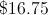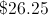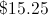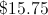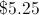Correct answer:Explanation: To find the part from the whole, multiply the "whole" by the percent (change the percent into a decimal first).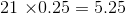The discount is$5.25.  Subtract the discount from the original price.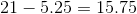.

So the t-shirt is on sale for \$15.75.

### Example Question #4 : How To Find The Part From The Whole

Sara and David bake a pie and cut it into 12 equal slices. Sara eatsof the pie, and David has 1 slice before givingof the original pie to his neighbor. How many slices of pie are left?Explanation:

Sara's portion is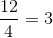While David's portion is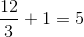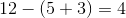### Example Question #5 : How To Find The Part From The Whole

What is 43% of 82? Round to the nearest tenths place.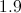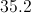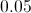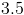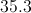Explanation:

To find the part from the whole, you will need to multiply the whole (82) by the percent. In order to do this, first change the percent to a decimal.Then, multiply the decimal and the whole.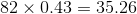43% of 82 is 35.3, rounded to the nearest tenth.

### Example Question #6 : How To Find The Part From The Whole

Fred wrote down a number greater than 11 and less than 15. When Susan tried to guess it, he told her it was greater than 13 and less than 17. What is the number?

11

14

12

16

14

Explanation:

Because Fred's number is between 11 and 15, it could be 12, 13, or 14. Later he tells Susan it is greater than 13 and less than 17, so it can only be 14, since he did not change his number.

### Example Question #3 : How To Find The Part From The Whole

What is 65% of 3.75?  Round to the nearest hundredths place.

2.4

2.44

2.438

2.4375

2.44

Explanation:

To find the part from the whole, you will need to multiply the whole (3.75) by the percent. In order to do this, first change the percent to a decimal (65% becomes 0.65).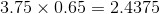Then you must round to the nearest tenths place: 2.4375 becomes 2.44.

Therefore, 65% of 3.75 is 2.44.

### Example Question #7 : How To Find The Part From The Whole

What is four-sevenths of 490?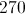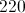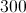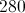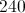Explanation:

Multiply the fraction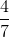by 490, putting 490 over 1 and cross-cancelling: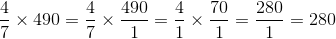### Example Question #8 : How To Find The Part From The Whole

What is nine-thirteenths of 234?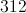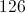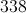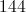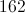Explanation:

Multiply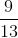by 234, rewriting and cross-cancelling: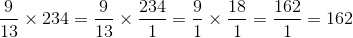### Example Question #4 : How To Find The Part From The Whole

A pizza contains 12 slices. Becky eats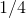of the pizza. How many slices does she eat?

4

6

3

5

3

Explanation:

Becky eatsof a pizza, which contains 12 pieces. You can find the total number that she eats by multiplying the fraction by the whole: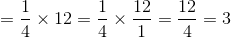← Previous 1 3 4 5 6